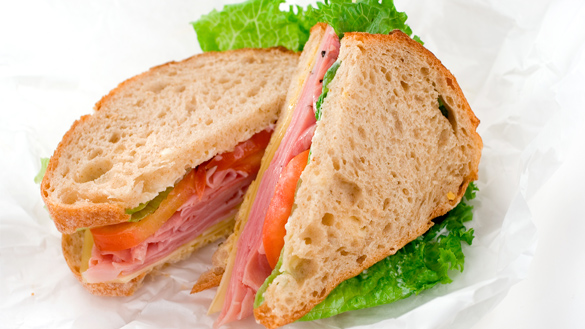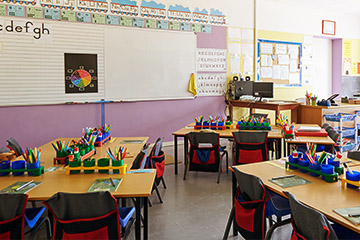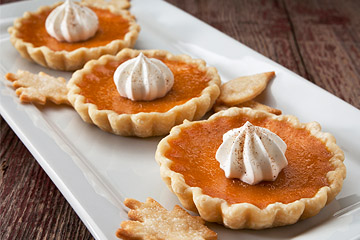# Minds On

## What does it mean to share?

One of the first things we learn is how important it is to share.

We share for fun when we share our toys with our friends.

We share to help when we share our lunch when a friend forgets theirs.What are some things you have shared?

When we share equally, it means that everyone gets the same or equal amounts. In math, we call this fair share.

## Solving fair share problems

Solve the following fair share problems using different representations.

Question 1

Max picked 20 apples from the apple tree. Max would like to share these apples with 4 friends. Max puts an equal number of apples in 4 baskets.

Using pictures, numbers and words show how Max shared these apples.This is an example of sharing more items with fewer groups.

Question 2

Jane has 2 brownies to share with their 4 friends.

What fraction of the brownies does each person get?This is an example of sharing fewer items with more groups.

When we equally share many items with fewer groups, the answer is a whole number.

When we equally share fewer items with more groups, the answer is a fraction.

### What is a fraction?

Let’s explore the following Homework Zone: Mathematics segment entitled “Parts of a Fraction” to learn about the different parts of a fraction.

What do fractions and fair sharing have in common? They both represent division.

In this lesson, we are going to learn to solve and compare fair share word problems using whole numbers and fractions.

# Action

## Equal groups in fair share problems

We use division to separate units into equal groups. In every fair share problem, we have to find how many are in each group OR how many groups there are.

## How many are in each group?

When you know how many groups there are, you can find how many are in each group.

For example:

There are 15 puppies napping in 3 puppy beds. How many puppies are in each bed?We can use pictures to help us solve the fair share problem.

#### Solution

We drew 3 boxes to represent the puppy beds. Next, we used circles to represent the puppies. We drew one circle in each box at a time until we had drawn 15 circles. Then, we counted how many circles were in each box. There were 5 circles in each box. So we know that 5 puppies slept in each bed.

## How many groups are there?

When you know how many units there are, you can find how many groups you need for a fair share.

For example:

There are 18 hamsters. 3 hamsters can fit in a play park.

How many play parks are needed for the hamsters?We can use pictures to help us solve the fair share problem.

#### Solution

We drew 18 diamonds to represent 18 hamsters. Then, we circled 3 diamonds at a time to represent the play park cage until all the diamonds were in a circle. Next, we counted the number of circles. There were 6 circles.

We knew that 6 play parks were needed.Student Success

### Think-Pair-Share

Use different representations to solve the problem.

#### Class Party!

Your class has won a party for reading the most books during the month of March. The principal has given your teacher a budget of $50.00 to spend on the party. Can you help the teacher decide what to buy? There are 20 students in your class, so you must make sure there is an equal share for everyone.Juice box 5 for$2Popcorn
50 cents eachPizza with 8 slices
$4 eachBoard game$5 each

Use a variety of representations to show your thinking.

Note to teachers: See your teacher guide for collaboration tools, ideas and suggestions.

## Solving fair share problems with fractions

A fraction shows equal parts of a whole object or set

How can you solve a fair share problem when the answer is not a whole number?

For example:

4 friends will share three chocolate bars equally. How much chocolate will each friend get?We can use pictures to help us solve the fair share problem.

#### SolutionWe know there are 4 friends and only 3 chocolate bars, so there are not enough to give each friend 1 whole. Hence, we know the answer will include fractions.

We used rectangles to represent the 3 chocolate bars. Then, we divided the rectangles into 4 equal parts. We can say each chocolate bar has a total of 4 parts. We numbered each part to represent 1 part for each friend. Then, we counted how many parts each friend gets.

Since there are 3 of each number, we know each friend gets 3 parts of the chocolate bar.

We can show this as a fraction: $\frac{3}{4}$.

## Practice

Model each situation in your notebook. Use a variety of representations to show your thinking.

##### Problem # 1

There are 28 books that must be returned to the library. Your teacher has asked four volunteers to help and would like each student to carry an equal number of books. How many books will each student carry? Use a method of your choice to show your thinking.

##### Problem # 2

Mr. Sanoor has 24 desks in their classroom. How many ways can Mr. Sanoor arrange the desks in their classroom so that each row has an equal number of desks? Use a division sentence for each possible way the desks can be arranged.

##### Problem #3A baker has 6 pumpkin pies to share with 6 of her friends. If the pies are shared equally, how many pumpkin pies will each friend get? What if the baker had 12 friends and wanted to share the 6 pies equally? Use a method of your choice to show your thinking.

Which part of the problem was the most challenging to solve? Explain your thinking.

What strategy did you use to help you solve each part of the question?

# Consolidation

## Recall the following practice questions

Problem # 1

There are 13 students in art class. Each student needs a paint brush. Paintbrushes come in packs of 2. How many packs of paint brushes should the teacher buy? How many paint brushes will be left over? Represent the exact number of packs of paint brushes needed as a fraction.Problem # 2

Mr. Sanoor has 24 desks in his classroom. How many ways can Mr. Sanoor arrange the desks in his classroom so that each row has an equal number of desks? Use a division sentence for each way he can arrange the desks.Problem # 3

Baker #1 has 3 pumpkin pies to share with 6 of their friends. How much pumpkin pie will each friend get? What if Baker #1 has 8 friends? What if Baker #1 has 10 friends? For each, show how much pie the friends would get.Choose one question to solve. Remember to explain your thinking in your notebook.

When you explain your thinking, remember to:

• present your ideas in order so they make sense
• use math language

Choose one question to model using a method of your choice. Remember to explain your thinking as you present your solution. Be sure to present your ideas in order so that they make sense and use appropriate math language.

## Reflection

How do you feel about what you have learned in this activity?  Which of the next four sentences best matches how you are feeling about your learning? Press the button that is beside this sentence.